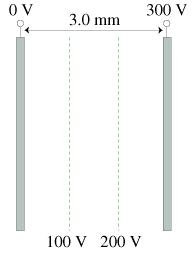# Problem: a. What is the electric field strength inside the capacitor?b. What is the potential energy of a proton at the midpoint of the capacitor?

###### FREE Expert Solution

a. Electric field strength:

$\overline{){\mathbf{E}}{\mathbf{=}}\frac{\mathbf{V}}{\mathbf{d}}}$

87% (463 ratings)###### Problem Detailsa. What is the electric field strength inside the capacitor?

b. What is the potential energy of a proton at the midpoint of the capacitor?